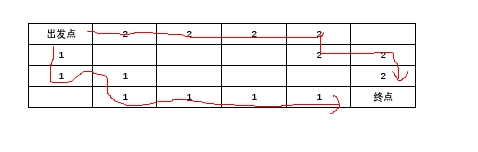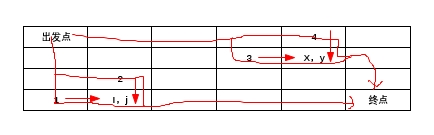# 第六届河南省程序设计大赛——D 探 寻 宝 藏（双向动态规划）

## 题目描述:

Dr.Kong希望他的机器人卡多尽量多地带出宝物。请你编写程序，帮助Dr.Kong计算一下，卡多最多能带出多少宝物。

## 输入描述:

<span style="color:#000000">第一行： K     表示有多少组测试数据。

【约束条件】
2≤k≤5      1≤M, N≤50     0≤Aij≤100    (i=1,….,M; j=1,…,N)

</span>

## 输出描述:

<span style="color:#000000">对于每组测试数据，输出一行：机器人卡多携带出最多价值的宝物数</span>

## 样例输入:

2
2 3
0 10 10
10 10 80
3 3
0 3 9
2 8 5
5 7 100


## 样例输出:

120
134

1、从左上角搜索到右下角，再从右下角反向搜索到左上角————找到两个不重复的从左上角到右下角的路径（如下图）2、创建用于动态规划的数组 dp[i][j][x][y]，其中ij确定的是第一条路径的坐标，xy确定的是第二条路径的坐标

3、每一个ij，xy都有可能是左方和上方转移过来的（如下图）4、其中（i，j）点可能是从1或者2号位置转移过来的，（x，y）点可能是从3或者4位置转移过来的，所以当1号路线和2号路线同时向右下进发一个位置（i，j）（x，y）时，有四种可能，有可能是从13位置转移过来的，也有可能是14，或者23或者24

ac代码：

#include<iostream>
#include<cstring>
#include<cstdio>
#include<algorithm>
#include<cmath>
using namespace std;
int k,n,m,ai,dp;
void dpnm(){
for(int i=1;i<=m;i++){
for(int j=1;j<=n;j++){
for(int x=1;x<=i;x++){
for(int y=1;y<=n;y++){
if(i==x&&j==y||i+j!=x+y)continue;   //当(i,j) (x,y)点相同或者走的步数不同时，跳过
int t1=dp[i-1][j][x-1][y],t2=dp[i-1][j][x][y-1],t3=dp[i][j-1][x-1][y],t4=dp[i][j-1][x][y-1];   //四个子位置的值
dp[i][j][x][y]=max(dp[i][j][x][y],max(max(max(t1,t2),t3),t4)+ai[i][j]+ai[x][y]);   //找到其中最大值并加上 (i,j) (x,y)两个位置本身含有的值
}
}
}
}
}
int main()
{
cin>>k;
while(k--){
cin>>m>>n;
fill(ai,ai+52*52,0);
fill(dp,dp+52*52*52*52,0);    //两个数组必须初始化为0；
for(int i=1;i<=m;i++){    //最好总1开始输入，
for(int j=1;j<=n;j++){
cin>>ai[i][j];
}
}
dpnm();
cout<<dp[m][n-1][m-1][n]+ai[m][n]<<endl;
}
return 0;
}

#include<iostream>
#include<cstdio>
#include<algorithm>
#include<cstring>
#include<cmath>
using namespace std;
int k,n,m,book,ai,maxsum=0;
void fandfs(int x,int y,int sum1){
book[x][y]=1;
if(x==0&&y==1&&sum1>maxsum)maxsum=sum1;
if(x==1&&y==0&&sum1>maxsum)maxsum=sum1;
if(x-1>=0&&!book[x-1][y]){
fandfs(x-1,y,sum1+ai[x-1][y]);
}
if(y-1>=0&&!book[x][y-1]){
fandfs(x,y-1,sum1+ai[x][y-1]);
}
book[x][y]=0;
return ;
}
void dfs(int x,int y,int sum1){
book[x][y]=1;
if(x==m-1&&y==n-1){
fandfs(x,y,sum1);
}
if(x+1<m&&!book[x+1][y]){
dfs(x+1,y,sum1+ai[x+1][y]);
}
if(y+1<n&&!book[x][y+1]){
dfs(x,y+1,sum1+ai[x][y+1]);
}
book[x][y]=0;
return ;
}
int main()
{
cin>>k;
while(k--){
fill(book,book+51*51,0);
cin>>m>>n;
for(int i=0;i<m;i++){
for(int j=0;j<n;j++){
cin>>ai[i][j];
}
}
dfs(0,0,ai);
cout<<maxsum<<endl;
maxsum=0;
}
return 0;
}

05-16668
12-0452511-30
05-28450
04-284476
10-03258
03-18146
07-182342
12-2289